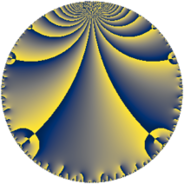# Properties

 Label 38.5.dLevel $38$ Weight $5$ Character orbit 38.d Rep. character $\chi_{38}(27,\cdot)$ Character field $\Q(\zeta_{6})$ Dimension $16$ Newform subspaces $1$ Sturm bound $25$ Trace bound $0$

# Related objects

## Defining parameters

 Level: $$N$$ $$=$$ $$38 = 2 \cdot 19$$ Weight: $$k$$ $$=$$ $$5$$ Character orbit: $$[\chi]$$ $$=$$ 38.d (of order $$6$$ and degree $$2$$) Character conductor: $$\operatorname{cond}(\chi)$$ $$=$$ $$19$$ Character field: $$\Q(\zeta_{6})$$ Newform subspaces: $$1$$ Sturm bound: $$25$$ Trace bound: $$0$$

## Dimensions

The following table gives the dimensions of various subspaces of $$M_{5}(38, [\chi])$$.

Total New Old
Modular forms 44 16 28
Cusp forms 36 16 20
Eisenstein series 8 0 8

## Trace form

 $$16q - 12q^{3} + 64q^{4} - 18q^{5} - 16q^{6} + 72q^{7} + 352q^{9} + O(q^{10})$$ $$16q - 12q^{3} + 64q^{4} - 18q^{5} - 16q^{6} + 72q^{7} + 352q^{9} - 84q^{11} + 450q^{13} + 288q^{14} - 390q^{15} - 512q^{16} + 606q^{17} - 306q^{19} - 288q^{20} - 2160q^{21} - 1680q^{22} - 54q^{23} + 128q^{24} - 434q^{25} + 1344q^{26} + 288q^{28} - 4914q^{29} + 2752q^{30} + 7890q^{33} - 1536q^{34} + 2328q^{35} - 2816q^{36} + 1344q^{38} + 7620q^{39} - 1692q^{41} + 2080q^{42} - 7402q^{43} - 336q^{44} - 16720q^{45} + 3198q^{47} + 768q^{48} + 24816q^{49} + 10710q^{51} + 3600q^{52} + 3870q^{53} - 16q^{54} - 13588q^{55} + 3702q^{57} - 1728q^{58} - 18288q^{59} - 3120q^{60} - 6522q^{61} - 6144q^{62} - 15676q^{63} - 8192q^{64} + 4960q^{66} - 30168q^{67} + 9696q^{68} + 15360q^{70} + 35874q^{71} + 5376q^{72} - 8080q^{73} - 9120q^{74} + 480q^{76} + 34560q^{77} - 46560q^{78} - 30738q^{79} - 1152q^{80} - 30920q^{81} + 6720q^{82} - 1476q^{83} + 33626q^{85} + 288q^{86} + 113100q^{87} + 19782q^{89} + 44256q^{90} - 34260q^{91} + 432q^{92} - 4272q^{93} - 23706q^{95} + 2048q^{96} - 9936q^{97} + 12672q^{98} + 3848q^{99} + O(q^{100})$$

## Decomposition of $$S_{5}^{\mathrm{new}}(38, [\chi])$$ into newform subspaces

Label Dim. $$A$$ Field CM Traces $q$-expansion
$$a_2$$ $$a_3$$ $$a_5$$ $$a_7$$
38.5.d.a $$16$$ $$3.928$$ $$\mathbb{Q}[x]/(x^{16} - \cdots)$$ None $$0$$ $$-12$$ $$-18$$ $$72$$ $$q+(-\beta _{5}+\beta _{9})q^{2}+(-1+\beta _{3})q^{3}+8\beta _{7}q^{4}+\cdots$$

## Decomposition of $$S_{5}^{\mathrm{old}}(38, [\chi])$$ into lower level spaces

$$S_{5}^{\mathrm{old}}(38, [\chi]) \cong$$ $$S_{5}^{\mathrm{new}}(19, [\chi])$$$$^{\oplus 2}$$